American Journal of Applied Chemistry
Volume 3, Issue 3, June 2015, Pages: 124-133

Synthesis and Sorption Studies of Lead (II) on Zn/Fe Layered Double Hydroxide

Ayawei N.1, Inengite A. K.1, Wankasi D.1, 2, Dikio E. D.1, 2

1Department of Chemical Sciences, Niger Delta University, Wilberforce Island, Bayelsa State, Nigeria

2Applied Chemistry and Nanoscience Laboratory, Department of Chemistry, Vaal University of Technology, Vanderbijlpark, South Africa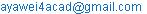(Ayawei N.)

Ayawei N., Inengite A. K., Wankasi D., Dikio E. D.. Synthesis and Sorption Studies of Lead (II) on Zn/Fe Layered Double Hydroxide. American Journal of Applied Chemistry. Vol. 3, No. 3, 2015, pp. 124-133. doi: 10.11648/j.ajac.20150303.15

Abstract: Zn/Fe-CO3 layered double hydroxide was synthesis by co-precipitation method for the adsorption of lead ions in aqueous solution. The synthesized layered double hydroxide (Zn/Fe-CO3) was then characterized by Powder X-ray Diffraction (PXRD), Fourier Transform Infrared (FT-IR) and SEM/EDX analysis. The experimental data fitted both Langmuir and Freundlich isotherms with regression correlation coefficient values of 0.9999 and 0.999 respectively. The essential thermodynamic parameters of DHo, DSo, DHx and Eawere calculated to be -4.8327KJ/mol, 12.8J/molK, 13.3KJ/mol and -1.948KJ/mol, thus showing the exothermic nature of the process and the randomness of the system. The low activation energy (Ea) value is consistent with physical adsorption. The results also fitted zero-order kinetic, first-order kinetic and pseudo-second order models.

Keywords: Thermodynamics, Freundlich Isotherm, Kinetics, Layered Double Hydroxide, Langmuir Isotherm

1. Introduction

Many industrial wastewaters contain dissolved metals as a result of their manufacturing processes. Included among them are mercury, lead, cadmium, silver, copper and chromium . Unlike organic pollutants, heavy metals are non-biodegradable contaminants and poses serious health and environmental hazards and removal of these wastes is a matter of must for government all over the world .

Among the different heavy metals, lead is one of the common and most toxic pollutants released into the natural waters from various industrial activities such as metal plating, oil refining and battery manufacturing . Lead ions are taken into body via inhalation, ingestion and/or skin adsorption. As a result when the body is exposed to lead, it can act as a cumulative poison.

Lead can replace calcium, which is an essential mineral for strong bones and teeth, while play important role in sympathetic actions of nerve and blood vessel for normal functioning of nervous system . The high level of lead damages cognitive development especially in children. Lead accumulates mainly in kidney, bones, brain and muscles and in the case of acute intoxication damage central nervous function, the cardiovascular and gastrointestinal (GI) systems, lungs, kidney, liver, endocrine glands and bones [5-6]. It also acts as an enzyme inhibitor in body, e.g. replaces essential element zinc from heme enzymes [7-8]. In drinking water maximum allowable limit of total Pb2+ of 50 gL-1 is considered safe by the World Health Organization, whereas less than 15 gL-1 is adopted by the United States Environmental Protection Agency .To avoid health hazards it is essential to remove these toxic heavy metals from wastewater before its disposal .

The traditional methods, for the treatment of lead and other toxic heavy metal contaminated wastewaters include complexation, chemical oxidation or reduction, solvent extraction, chemical precipitation, reverse osmosis, ion exchange, filtration, membrane processes, evaporation and coagulation. Besides the classical wastewater treatment techniques, adsorption of heavy metals is the most promising separation and purification method because this technique has significant advantages including high efficiency in removing very low levels of heavy metals from dilute solutions, easy handling, high selectivity, lower operating cost, minimum production of chemical or biological sludge and regeneration of adsorbent .

Layered nanomaterials are getting lots of attention due to their unique structural flexibility, which is useful for the development of new hybrid materials with controlled functionality . The layered double hydroxide (LDH) is also known as hydrotalcite-like material or anionic clay, are the large group of natural or synthetic materials that are layered, containing the hydroxide of two or more different kinds of metal cations and possessing an overall positive charge, which neutralized by the incorporation of exchangeable anion . The structure of LDH is closely related to brucite-like layer, Mg(OH)2, where Mg2+ is octahedrally surrounded by six OH-and share edges to form infinite sheet . Some of the divalent ions are replaced by trivalent ions, resulting in positively charge sheet and compensated by anions in the interlayer galleries along with the water molecules [15-17].

The general formula that represents this class of materials is [M2+1-xM3+x(OH)2]x+ (An-x/n).mH2O, where M2+ is divalent cation (Ca2+ , Mg2+ , Zn2+ , Co2+ , Ni2+ , Cu2+ , Mn2+ ), M3+ is trivalent cation (Al3+ , Cr3+ , Fe3+, Co3+ , Ni3+, Mn3+) and An- is an interlayer anion (Cl-, NO3-, ClO4-, CO3-, SO42- and other inorganic anions). The x value is the charge density for the molar ratio M3+/(M 2+ +M3+). The monovalent anions such as NO3- and Cl- within the interlayer gallery space can be easily replaced by desired anions. The structure of LDH forms two-dimensional crystals consisting of thin crystalline layered that stacked by van der Waals and/or weak electrostatic interaction; thus various guest anions can be inserted into the LDH interlayer galleries. This material is two dimensional type layered structure consisting of thin crystalline layers with a thickness of a few nanometers 

LDHs have anionic exchange capacity and the ability to capture organic and inorganic anions make them almost unique as inorganic materials and are generally termed environment scavengers. The application of some LDHs as sorbents for the removal of some heavy metals has been studied [19-20]. The results indicated that indeed layered double hydroxides are a potential remedy to heavy metals pollution.

The aim of the present work is to investigate the uptake of lead by Zn/Fe layered double hydroxide under the different experimental conditions with a view to using the LDH as potential adsorbent for environmental remediation.

2. Experimental

2.1. Synthesis of Zn/Fe-CO3

Carbonate form of Zn-Fe-CO3 was synthesized by co-precipitation method. A 50 ml aqueous solution containing 0.3 M Zn (NO3)2.6H2O and 0.1 M Fe (NO3)3.9H2O with Zn/Fe ratios 3:1, was added drop wise into a 50 ml mixed solution of (NaOH (2M) + Na2CO3 (1M) with vigorous stirring and maintaining a pH of greater than 10 at room temperature. After complete addition which last between 2 hours 30 minutes to 3 hours, the slurry formed was aged at 60°C for 18 hours. The products were centrifuged at 5000 rpm for 5 minutes, with distilled water 3-4 times and dried by freeze drying.

2.2. Characterization of Zn/Fe-CO3

FESEM/EDX was obtained using Carl Zeiss SMT supra 40 VPFESEM Germany and incapentaFET x 3 EDX, Oxford. It was operated at extra high tension (HT) at 5.0 kV and magnification at 20000X. FESEM uses electron to produce images (morphology) of samples and was attached with EDX for qualitative elemental analysis.

FTIR spectrum was obtained using a Perkin Elmer 1725X spectrometer. where samples will be finely ground and mixed with KBr and pressed into a disc. Spectrums of samples were scanned at 2 cm-1resolution between 400 and 4000 cm-1.

X-ray diffraction (XRD) pattern of the sample was characterized by using a Shimadzu XRD-6000 diffractometer, with Ni-filtered Cu-Kα radiation ( λ = 1.54 A°) at 40 kV and 200 mA. Solid samples were mounted on alumina sample holder and basal spacing (d-spacing) was determined via powder technique. Samples scan were carried out at 10-60°, 2o/ min at 0.003° steps

2.3. Preparation of Metal Ion Solution

The adsorbate Pb2+was procured from Zayo-Sigma Chemical Ltd. Jos, Nigeria. The stock solution of Pb2+ was prepared by dissolving a required amount of Pb(NO3)2 in doubly distilled water at room temperature. The experimental solutions were obtained by diluting a stock solution of Pb3+with double distilled water to the desired concentration.

A 0.2g each of the powder sample was collected and weighed using an electronic weighing balance, the weighed sample was placed in three (3) pre-cleaned test tube. Ten ml of each metal ion solution with standard concentration of 0.13g.L-1, 0.25g.L-1 and 0.38g.L-1 which was made from spectroscopic grade standards of lead ion (Pb2+) [from lead nitrate: Pb(NO3)2] were added to each test tube containing the weighed sample and equilibrated by rocking (agitation) for 30 minutes and then centrifuged at 2500rpm for 5 minutes and decanted. The supernatants were stored for lead ion (Pb2+) analysis as stated in metal analysis.

A 0.2g each of the powder sample was weighed using an electronic weighing balance and placed in three (3) pre-cleaned test tube. 10ml of the metal ion solution with standard concentration of 0.35g/L which was made from spectroscopic grade standard of lead ion (Pb2+) [from lead nitrate: Pb(NO)3)2] was added to each test tube containing the weighed sample and equilibrated rocking (agitation) for each time intervals of 10, 20 and 30 minutes respectively. The powered sample suspension were centrifuged for 5 minutes at 2500rpm and decanted. The supernatants were stored for lead ion (Pb2+) analysis as stated in metal analysis.

A 0.2g each of the powder sample was collected and weighed using an electronic weighing balance; the weighed sample was placed in three (3) pre-cleaned tubes. 10ml of the metal ion solution with standard concentration of 0.38g/L which was made from spectroscopic grade standard of lead ion (Pb2+) [from lead nitrate: Pb(N0)3)2] was added to each test tube containing the weighed sample and equilibrated rocking (agitation) for 30 minutes at temperatures of 400C, 600C and 800C respectively using Gallenhamp water bath. This was immediately centrifuged at 2500rpm for 5 minutes and then decanted. The supernatant were stored for lead ion (Pb2+) analysis as stated in metal analysis.

3. Results and Discussions

Scanning electron microscope (SEM) and Energy dispersive spectroscope (EDS) images of as-synthesized layered double hydroxides are presented in figures (1-2). The images shows the surface morphology of the layered double hydroxides before and after adsorption studies. SEM image before adsorption studies, figure 1(a), shows a heterogeneous/rough surface with several pores whiles the image after adsorption studies, figure 1(b), show a smooth surface with several agglomeration of reacted hydroxide line. The smooth surface observed could be due to adsorbed metal ions filling the pores that existed before adsorption studies. The energy dispersive spectrograph before adsorption studies, figure 2(a), show the presence of metal ions used in the synthesis of the layered double hydroxides such as aluminium, sodium, and nickel and their percentage compositions. After adsorption studies, figure 2(b), the energy dispersive spectrograph, show the presence of lead ions adsorbed by the layered double hydroxide. The EDS also show that a chemical change has taken place during adsorption studies as observed in the elemental composition presented.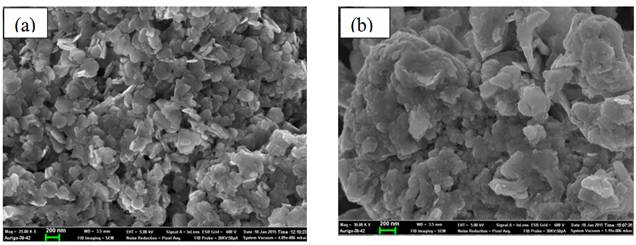Figure 1. Scanning Electron Microscope (SEM) micrograph of Zn/Fe-CO3 before (a) and after (b) adsorption studies.

3.1. EDX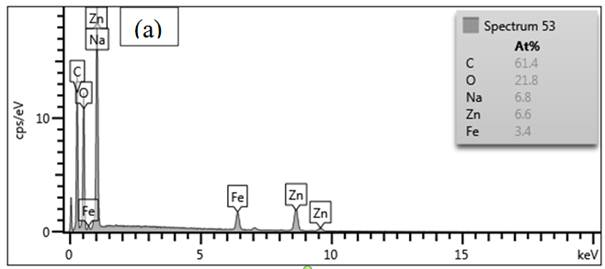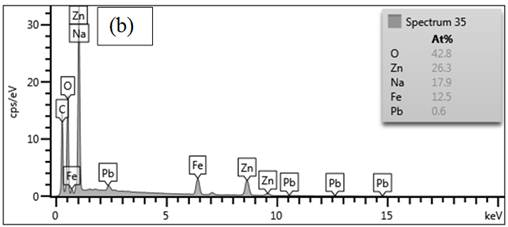Figure 2. Energy Dispersive Spectroscopy patterns of Zn/Fe-CO3 pre & post adsorption Energy Dispersive Spectroscopy.

3.2. FT-IR

As shown in Figure 3, the band near 3340 cm−1 corresponds to the vibration bands of hydroxyls (ѵOH). The bending mode of water molecules appears at 1639 cm−1. The sharp intense band at 1359 cm−1 is the antisymmetric stretching of interlayer carbonate and the band at 837 cm-1is due to M-O vibration. The band at 1048cm-1 is due to C-O stretching.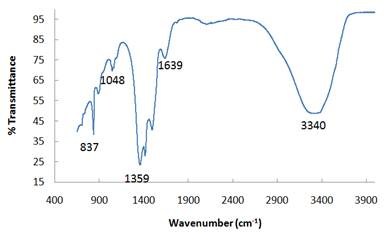Figure 3. Zn/Fe-C03 Fourier transform infrared spectroscopy.

3.3. XRD

Figure 4 shows XRD patterns of Zn-Fe-CO3-LDH prepared at differentZn2+/Fe3+ molar ratios of 2:1. XRD patterns exhibit two characteristic intense peaks of basal reflection of Zn-Fe-CO3-LDH which were located near 2𝜃 of 8.46oand 30.14ocorresponding to diffraction by (003) and (006) planes and d-spacing of 1.04 and 0.2911 respectively. These two peaks show the presence of an ordered layered structure.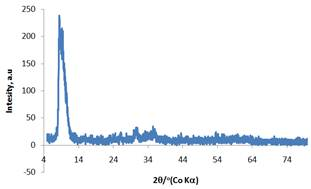Figure 4. Zn/Fe-C03X-ray powder diffraction.

In order to describe the adsorbate-adsorbent interaction, the isotherm data were analyzed by fitting them into Langmuir and Freundlich equations to find out the suitable model that may be used for design consideration.

The Langmuir adsorption mode is based on the assumption that maximum adsorption corresponds to saturated monolayer of solute molecules on the adsorbent surface. The linear form of Langmuir equation is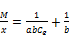(1)

Where X is the amount of Pb2+ adsorbed per mass M of layered double hydroxide, in mg/g a and b are the Langmuir constants obtained from the slope and intercepts of the plots.

The plot of M/X against 1/Ce (Figure 5) shows the adsorption obeys equation (2). The Langmuir constants a and b which are determined from the slope and intercept of the plot, are 0.957 and intercept 5.2 respectively. The R2value (0.9999) suggest that the adsorption follows Langmuir model.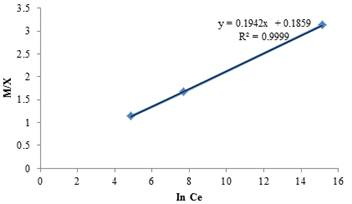Figure 5. Langmuir isotherm plot for Pb2+ onto layered double hydroxide.

The essential characters of Langmuir isotherm can be expressed in term of dimensionless constant separation factor RL given by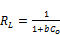(2)

Where Co (mg L-1) is the highest initial concentration of Pb2+ and b(L mg-1) is Langmuir constant.

The value of RL in the present investigation is found to be 0.713 showing the adsorption is favoured (0<RL<1) at the temperature studied.

The Freundlich isotherm considers multilayer adsorption with heterogeneous energetic distribution of active sites accompanied by interaction between adsorbed molecules.  The linear Freundlich isotherm is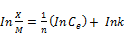(3)

Where, K is Freundlich constant, which indicates the relative adsorption capacity of the adsorbent and n is a measure of the adsorption intensity or surface heterogeneity (a value closer to zero represents a more heterogeneous surface).

The linear plot of InX/M against InCe, (Figure 6) shows that the adsorption of Pb2+ on layered double hydroxide also follows Freundlich isotherm model. Freundlich constant K and n were 2.425 and 0.778 respectively. The value of n>1 indicates favourable adsorption. Regression correlation coefficient (R2 = 0.999).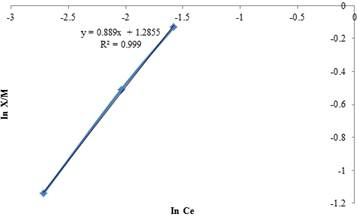Figure 6. Freundlich isotherm plot for the adsorption of Pb2+ onto layered double hydroxide.

Thermodynamic considerations of an adsorption process are necessary to conclude whether the process is spontaneous or not. The Gibb’s free energy change, ΔGo, is an indication of spontaneity of a chemical reaction and therefore is an important criterion for spontaneity. Both enthalpy (ΔHo) and entropy (ΔSo) factors must be considered in order to determine the Gibb’s free energy of the process. Reactions occur spontaneously at a given temperature if ΔGo is a negative quantity. The free energy of an adsorption process is related to the equilibrium constant by the classical Van’t Hoff equation (Equation 7):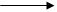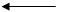(4)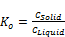(5)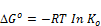(6)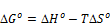(7)

The values of ∆Go were obtained from the application of equation (7). The negative values of ∆Go (Table 1) shows that the adsorption is highly favorable and spontaneous. It was also observed that the negative values of ∆Go decreases with increase in temperature, which indicate that the adsorption is preferable at low temperature, which was observed from the experimental data.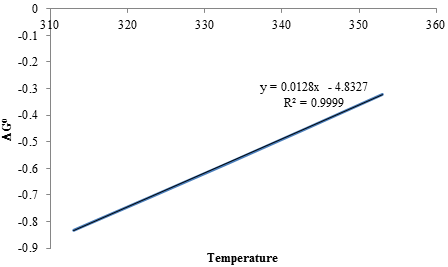Figure 7. Plot of DGo vs. Temperature for the adsorption of Pb2+ onto layered double hydroxide.

In order to further support the assertion that physical adsorption is the predominant mechanism, the values of activation energy (Ea) and sticking probability (S*) were estimated from the experimental data. They were calculated using modified Arrhenius type equation related to surface coverage (q) as follows: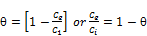(8)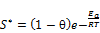(9)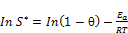(10)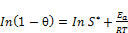(11)

The sticking probability S *, is a function of the adsorbate / adsorbent system under investigation, its value lies in the range 0<S *<1 for preferable process and is dependent on the temperature of the system. The q is the surface coverage, which can be calculated from equation 9. The values of Ea and S * can be calculated from slope and intercept of the plot of ln(1-q) versus 1/T respectively (Figure 11 & Table 1). The values of Ea was found to be -1.948 kJ/mol -1 for the adsorption of Pb2+ onto the layered double hydroxide. The negative Ea value indicates that low temperature or energy favours the sorption and the process is exothermic. The sticking probability value (0.42) was less than 1, which indicates that the probability of the Pb2+ ions to stick on surface of layered double hydroxide is very high as S*<< 1, this value confirm that, the sorption process is physisorption .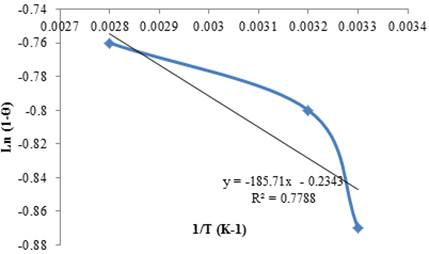Figure 8. Plot of In(1-q) vs. reciprocal of time for the adsorption of Pb2+ onto layered double hydroxide.

The isosteric heat of adsorption (DHx) provides information about surface energetic heterogenicity and is a critical design variable in estimating the performance of an adsorptive separation process . It can be calculated using a Freundlich isotherm is expressed by the following Claussius–Clapeyron equation (Equation. 14).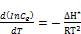(12)

where, Ce is the equilibrium adsorbate concentration in the solution (mg.L-1), ΔHx is the isosteric heat of adsorption (kJ mol-1), R is the ideal gas constant (8.314 J.mol-1K-1), and T is temperature (K). Integrating the above equation, assuming that the isosteric heat of adsorption is temperature independent, gives the following equation: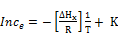(13)

where K is a constant.

The isosteric heat of adsorption is calculated from the slope of the plot of ln Ce versus 1/T different amounts of adsorbate onto adsorbent.

The magnitude of ΔHx (13.3 kJ mol-1)as extrapolated from the slope in figure 12 gives information about the adsorption mechanism as chemical ion-exchange or physical sorption. For physical adsorption, ΔHx should be below 80 kJ mol-1 and for chemical adsorption it ranges between 80 and 400 kJ.mol-1 [18,19,20].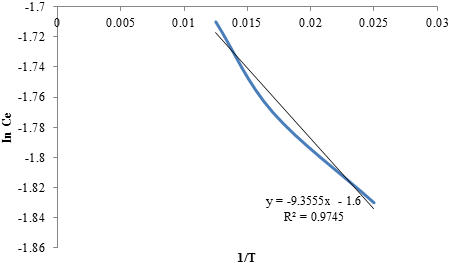Figure 9. Plot of In Ce vs. reciprocal of time for the adsorption of Pb2+ onto layered double hydroxide.

Table 1. Thermodynamic Parameters of the Adsorption of Lead onto Layered Double Hydroxide.

 T,K DGo, KJ/mol DHo, KJ/mol DSo, J/mol K Ea, KJ/mol DHx, KJ/mol 313 -0.833 -4.833 12.8 -1.948 13.3 333 -0.581 353 -0.322

Zero-order kinetic, first-order kinetic and Pseudo second order models were applied to test the experimental data and explain the kinetics of the layered double hydroxide adsorption process.

Zero-order kinetic model,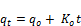(14)

where, ko (min-1) is the rate constant,

qo (mg g-1) is the amount of Pb2+ adsorbed on surface at equilibrium,

qt (mg g-1) is the amount of Pb2+ adsorbed on surface at time t (min).

The adsorption rate constant, ko and qo were calculated from the plot of qt vs. t, as shown in figure 10.

First-order kinetic model is given as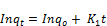(15)

where, k1 (min-1) is the rate constant,

qo (mg g-1) is the amount of Pb2+ adsorbed on surface at equilibrium,

qt (mg g-1) is the amount of Pb2+ adsorbed on surface at time t (min).

The adsorption rate constant, k1 and qo were calculated from the plot of In qt vs. t, as shown in figure 11.

The pseudo second order kinetic model is given by equation .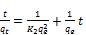(16)

where, k2 (g mg-1 min-1) is pseudo second order rate constant. The plot of t/qt vs t is shown in Figure 12 gives the values of qe and k2.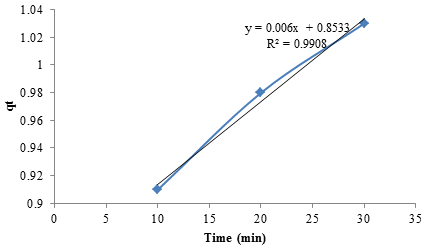Figure 10. Plot of qt vs. t for adsorption of Pb2+ onto layered double hydroxide.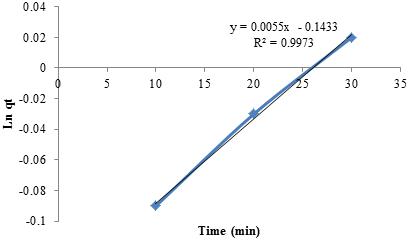Figure 11. Plot of In qt vs. t for adsorption of Pb2+ onto layered double hydroxide.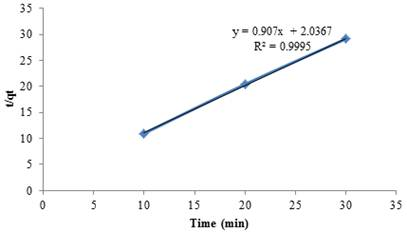Figure 12. Plot of t/qt vs. t for adsorption of Pb2+ onto layered double hydroxide.

The results from figures 10 - 12 indicate that the kinetic models were all linear.

4. Conclusion

The discharge of toxic heavy metals to our delicate and precious environment is a wide spread phenomenon. Adsorption readily provides an efficient option amongst other methods for the removal of these heavy metals. This study has shown that Zn/Fe-CO3 layered double hydroxide could be used as an adsorbent for the removal of heavy metal in aqueous media. The experimental results point to the fact that the adsorption ability of the layered double hydroxide could be enhanced by increasing the quantity used during adsorption process.

Reference

1. Abia A. A., Didi, O. B., Asuquo E. D. Modelling of Cd2+ sorption kinetics from aqueous solutions onto some thiolated agricultural waste adsorbents. J. Appl. Sci. (2006). 6:2549-2556.
2. Amarasinghe B. M. W. P. K., Williams R. A. Tea waste as a low cost adsorbent for the removal of Cu and Pb from wastewater. Chem, Eng (2007). J. 32: 299-309.
3. Teoh Y.P., Khan M.A., Choong T.S.Y. Kinetic and isotherm studies for lead adsorption from aqueous phase on carbon coated monolith, Chemical Engineering Journal, (2013), 217, 248–255.
4. Martins, R. J. E., and Boaventura, R. A. R. "Modelling of lead removal by an aquatic moss," Water Sci. Technol. (2011) 63(1), 136-142.
5. Dimple L. Adsorption of Heavy Metals: A review, International Journal of Environmental Research and Development (2014). 1: 41-48
6. Argun M. E., Dursun S., Ozdemir C., Karatas M. Heavy metal adsorption by modified oak sawdust: thermodynamics and kinetics. J. Hazard. Mater. B (2007). 141:77-85
7. Skerfving S., Gehardsson L., Schütz A., Strömberg U. Lead-biological monitoring of exposure and effects. J. Trace Elem. Exp. Med (1998). 11: 289-301.
8. Soylac M., Elci L., Akkaya Y., Dogan M., On-line preconcentration system for determination of lead in water and sediment samples by flow injection-flame atomic absorption spectrometry AnalLett (2002). 35: 487-499.
9. Raungsomboon S., Chidthaisong A., Bunnag B., Inthron D., Harvey, N.W., Removal of lead Pb+2 by the CyanobacteriumGloeocapsa sp. Bioresour. Technol (2007). 99, 5650-5658.
10. Ayawei N., Horsfall M. and Spiff A. I. Rhizophora mangle waste as adsorbent for metal ions removal from aqueous solution, European Journal of Scientific Research (2005). Vol. 9. No. 1: pp 6-21.
11. King P., Rakesh N., Beenalahari S., Prasamna Y. K., Prasad V. S. R. K.,. Removal of lead from aqueous solution using Syzygiumcumini L.: equilibrium and kinetic studies, J. Hazard. Mater (2007).142: 340-347.
12. Oh J. M., Biswick T. T., Choy J. H., Layered material for green materials, J. Mater. Chem (2009). 19: 2553-2563.
13. Braterman P. S., Xu Z.P., Yarberry F., Layered Double Hydroxide, in: S. M.Aubach, K. A. Carrado, and P.KDutta (Eds.), Handbook of layered materials, Marcel Dakker., New York, (2004). pp. 373-450.
14. Cavani F., Trifiro F., Vaccari A. Hydrotalcite-type anionic clays: preparation, properties and applications, Catal (1991). Today 11 173-301.
15. Tronto J., Sanchez K. C., Crepaldi E. L., Naal Z., Stanlei I. K., Valim J. B., Synthesis, characterization and electrochemical stidy of layered double hydroxide intercalated with 2-thiophenecarboxylate anions, J. Phys. Chem. Solid (2004). 65: 493-498.
16. Silion M., Hritcu D., Lisa G., Popa M. I., New hybrid materials based in layered double hydroxides and antioxidant compounds. Preparation, characterization and release kinetic studies, J. Porous Mater (2012).19: 267-276.
17. Sarijo S.H., Ghazali S.A.I.S.M., Hussien M.Z., Sidek N.J. Synthesis of nanocomposite 2-methyl-4-chlorophenoxyacetic acid with layered double hydroxide: physiochemical characterization and controlled release properties, J. Nanopart. Res (2013). 15: 1356-1365.
18. Ahmed A. A. Ali, Abidin Talib Z., and Hussein M. Z. B., "ESR spectra and thermal diffusivity of ZnAl layered double hydroxide," Journal of Physics and Chemistry of Solids, vol. 73, no. 1, pp. 124–128, 2012.
19. Ayawei, N., Ekubo, A. T., Wankasi, D., and Dikio, E. D. Adsorption Dynamics of Copper Adsorption by Zn/Al-CO3. IJACSA (2015). Vol. 3, Issue 1: 57 - 64.
20. Ayawei, N., Ekubo, A. T., Wankasi, D., and Dikio, E. D. Equilibrium, Thermodynamic and Kinetic Studies of the Adsorption of Lead(II) on Ni/Fe Layered Double Hydroxide. Asian Journal of Applied Sciences (ISSN: 2321 – 0893)(2015). Volume 03 – Issue 02: 207-217.
21. Ayawei, N., Ekubo, A. T., Wankasi, D., and Dikio, E. D. Mg/Fe Layered double hydroxide for removal of lead (II): Thermodynamic, Equilibrium and Kinetic Studies. European Journal of Science and Engineering (2015).Vol. 3, No.1; 1-17.
22. Ayawei, N., Ekubo, A. T., Wankasi, D., and Dikio, E. D. "Synthesis and Application of Layered Double Hydroxide for the removal of Copper in Wastewater". International Journal of Chemistry (2015) . Vol. 7, No. 1: 122 - 132.

 Contents 1. 2. 2.1. 2.2. 2.3. 2.4. 3. 3.1. 3.2. 3.3. 3.4. 3.5. 3.6. 4.
Article ToolsAbstractPDF(4035K)